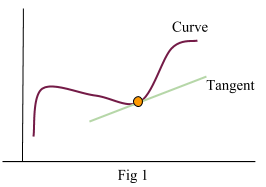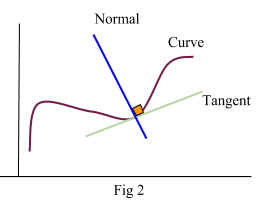# Tangents and Normals

## Summary

• A tangent is a line that just touches the curve but doesn’t go through it.
The gradient of a tangent = Gradient of a curve at that point
• A normal is a straight line perpendicular (at right angle 90°) to a curve.$Gradient\quad of\quad a\quad normal\quad =\quad \frac { -1 }{ (Gradient\quad of\quad a\quad curve\quad or\quad tangent\quad at\quad that\quad point) }$

We know that differentiation is the process that we use to find the gradient of a point on the curve. However, we can also find the gradient of a curve at a given point by drawing a tangent at that point (which is a straight line) and finding the gradient of that line which is equal to the gradient/derivative of the curve as well.

#### What is a Tangent?

As shown in Fig 1, a tangent just touches the curve but doesn’t go through it. The line intersects the curve at a point and the gradient of the line is equal to the derivative of the curve at the point.#### Example #1

Q. Find the equation of tangent to the curve y = (6 – x)(x + 3) at the point (3, 5)

Solution:

Write the equation in the form that we can differentiate:$y\quad =\quad (6\quad -\quad x)(x\quad +\quad 3)$$=\quad { -x }^{ 2 }\quad +\quad 3x\quad +\quad 18$

Now differentiate it:$\frac { dy }{ dx } \quad =\quad -2x\quad +\quad 3$

The gradient of the tangent is equal to gradient of the curve at point (3, 5):

At x=3$\frac { dy }{ dx } \quad =\quad -3$

Hence m(gradient) = -3 as well.

Now to find the equation of the tangent:$y\quad -\quad { y }_{ 1 }\quad =\quad m(x\quad -\quad { x }_{ 1 })$

Point$({ x }_{ 1 },\quad { y }_{ 1 })$  is (3, 5) and m = -3$y\quad -\quad 5\quad =\quad -3(x\quad -\quad 3)$$y\quad =\quad -3x\quad +\quad 14\quad \quad \quad \longrightarrow$  equation of tangent at point (3, 5)

#### What is a Normal?

As shown in Fig 2, A normal is a straight line perpendicular (at right angle 90°) to a curve or to the tangent of the curve at that point.If two lines, with gradients m1 and m2 are at right angles then$m1\quad \times \quad m2\quad =\quad -1$.

Therefore if the gradient of the tangent is m1 than the gradient of the normal is$m2\quad =\quad \frac { -1 }{ m1 }$

#### Example #2

Q. Find the equation of the normal to the curve$y\quad =\quad { x }^{ 3 }\quad -\quad 4x\quad +\quad 2$  at the point where x = 2.

Solution:

Firstly find the y coordinate at x=2:$y\quad =\quad { (2) }^{ 3 }\quad -\quad 4(2)\quad +\quad 2$$y\quad =\quad 2$

Now differentiate the equation of the curve to find the gradient of the tangent at:$\frac { dy }{ dx } \quad =\quad { 3x }^{ 2 }\quad -\quad 4$

At x=2$\frac { dy }{ dx } \quad =\quad 8$

Hence m1 = 8

Find m2:$m2\quad =\quad \frac { -1 }{ 8 }$

Equation of normal is$y\quad -\quad { y }_{ 1 }\quad =\quad m(x\quad -\quad { x }_{ 1 })$  where Point$({ x }_{ 1 },\quad { y }_{ 1 })$  is (2, 2) and$m\quad =\quad \frac { -1 }{ 8 }$.$y\quad -\quad 2\quad =\quad \frac { -1 }{ 8 } (x\quad -\quad 2)$$y\quad =\quad \frac { -1 }{ 8 } x\quad +\quad \frac { 9 }{ 4 } \quad \quad \quad \longrightarrow$    equation of normal at point (2, 2)

##### Reference
1. CGP AS-Level Edexcel Mathematics complete revision and practice book